### Lack-of-Fit Tests

Two goodness-of-fit tests can be requested from the PROBIT procedure: a Pearson’s chi-square test and a log-likelihood ratio chi-square test.

To compute the test statistics, you can use the AGGREGATE or AGGREGATE= option grouping the observations into subpopulations. If neither AGGREGATE nor AGGREGATE= is specified, PROC PROBIT assumes that each observation is from a separate subpopulation and computes the goodness-of-fit test statistics only for the events/trials syntax.

If the Pearson’s goodness-of-fit chi-square test is requested and the p-value for the test is too small, variances and covariances are adjusted by a heterogeneity factor (the goodness-of-fit chi-square divided by its degrees of freedom) and a critical value from the t distribution is used to compute the fiducial limits. The Pearson’s chi-square test statistic is computed as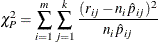where the sum on i is over grouping, the sum on j is over levels of response,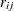is the frequency of response level j for the ith grouping,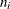is the total frequency for the ith grouping, and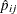is the fitted probability for the jth level at the ith grouping.

The likelihood ratio chi-square test statistic is computed as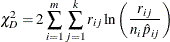This quantity is sometimes called the deviance. If the modeled probabilities fit the data, these statistics should be approximately distributed as chi-square with degrees of freedom equal to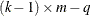, where k is the number of levels of the multinomial or binomial response, m is the number of sets of independent variable values (covariate patterns), and q is the number of parameters fit in the model.

In order for the Pearson’s statistic and the deviance to be distributed as chi-square, there must be sufficient replication within the groupings. When this is not true, the data are sparse, and the p-values for these statistics are not valid and should be ignored. Similarly, these statistics, divided by their degrees of freedom, cannot serve as indicators of overdispersion. A large difference between the Pearson’s statistic and the deviance provides some evidence that the data are too sparse to use either statistic.